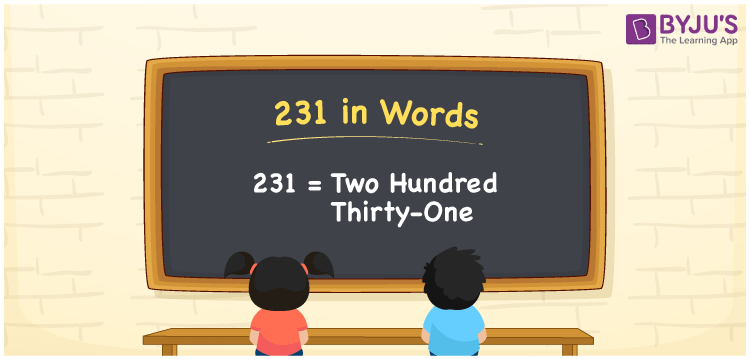# 231 in Words

In Mathematics, 231 in words is Two hundred thirty-one. Learning the number and its name is essential, as it helps to count the objects, measure distance and so on. For example, there are 231 kilometers to reach Akshaya’s home. This article discusses the procedure of writing the number 231 in words using the place value system.

 231 in Words: Two Hundred Thirty-one. Two Hundred Thirty-one in Numerical Form: 231.

## 231 in English Words## How to Write 231 in Words?

The place value table for the number is provided below.

 Hundreds Tens Ones 2 3 1

The expanded form of 231 is as follows:

= 2 × Hundred + 3 × Ten + 1 × One

= 2 × 100 + 3 × 10 + 1 × 1

= 200 + 30 + 1

= 231

= Two hundred thirty-one

Hence, 231 in words is two hundred thirty-one.

The number 231 is a natural number that exists between 230 and 232.

231 in words – Two hundred thirty-one

Is 231 an odd number? – Yes

Is 231 an even number? – No

Is 231 a perfect square number? – No

Is 231 a perfect cube number? – No

Is 231 a prime number? – No

Is 231 a composite number? – Yes

## Frequently Asked Questions on 231 in Words

Q1

### Write 231 in words.

231 in words is two hundred thirty-one.

Q2

### Simplify 200 + 31, and express it in words.

Simplifying 200 + 31, we get 231. Hence, 231 in words is two hundred thirty-one.

Q3

### Is 231 an even number?

No, 231 is not an even number.